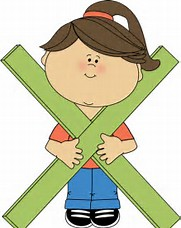Schools

We are doing two digit by one digit multiplication!3.6 The student will represent multiplication and division, using area, set, and number line models, and create and solve problems that involve multiplication of two whole numbers, one factor 99 or less and the second factor 5 or less.

We will be reviewing for math SOL's!

3.1 The student will a) read and write six-digit numerals and identify the place value and value of each digit; b) round whole numbers, 9,999 or less, to the nearest ten, hundred, and thousand; and c) compare two whole numbers between 0 and 9,999, using symbols (>, <, or = ) and words (greater than, less than, or equal to).
3.4 The student will estimate solutions to and solve single-step and multistep problems involving the sum or difference of two whole numbers, each 9,999 or less, with or without regrouping.
3.17 The student will a) collect and organize data, using observations, measurements, surveys, or experiments;
b) construct a line plot, a picture graph, or a bar graph to represent the data; and c) read and interpret the data represented in line plots, bar graphs, and picture graphs and write a sentence analyzing the data.
3.19 The student will recognize and describe a variety of patterns formed using numbers, tables, and pictures, and extend the patterns, using the same or different forms.